# High School Chemistry : Help with Orbital Diagrams and Theories

## Example Questions

### Example Question #1 : Help With Orbital Diagrams And Theories

Which of the following elements does not exist as a diatomic molecule?

Helium

Iodine

Hydrogen

Oxygen

Chlorine

Helium

Explanation:

A diatomic element is an element that will spontaneously bond with itself if it isn't already part of a compound. This is done to increase stability. The seven diatomic elements are hydrogen, oxygen, nitrogen, fluorine, chlorine, bromine, and iodine. Note that all of these except iodine are gasses at room temperature. Helium is a noble gas, which means it has a full valence shell. Helium does not need to bind to anything to increase its stability.

### Example Question #101 : High School Chemistry

How many valence electrons does a fluorine atom have?Explanation:

An atom's valence electrons are the electrons on the outermost shell surrounding the atom. For the first shell, the number of valence electrons can be at most 2. For all subsequent shells, the maximum is 8. To determine the number of valence electrons around a neutral atom, you match the atom with its group number. As you can see from the picture below, fluorine has a group number of 7. Therefore, it has 7 valence electrons.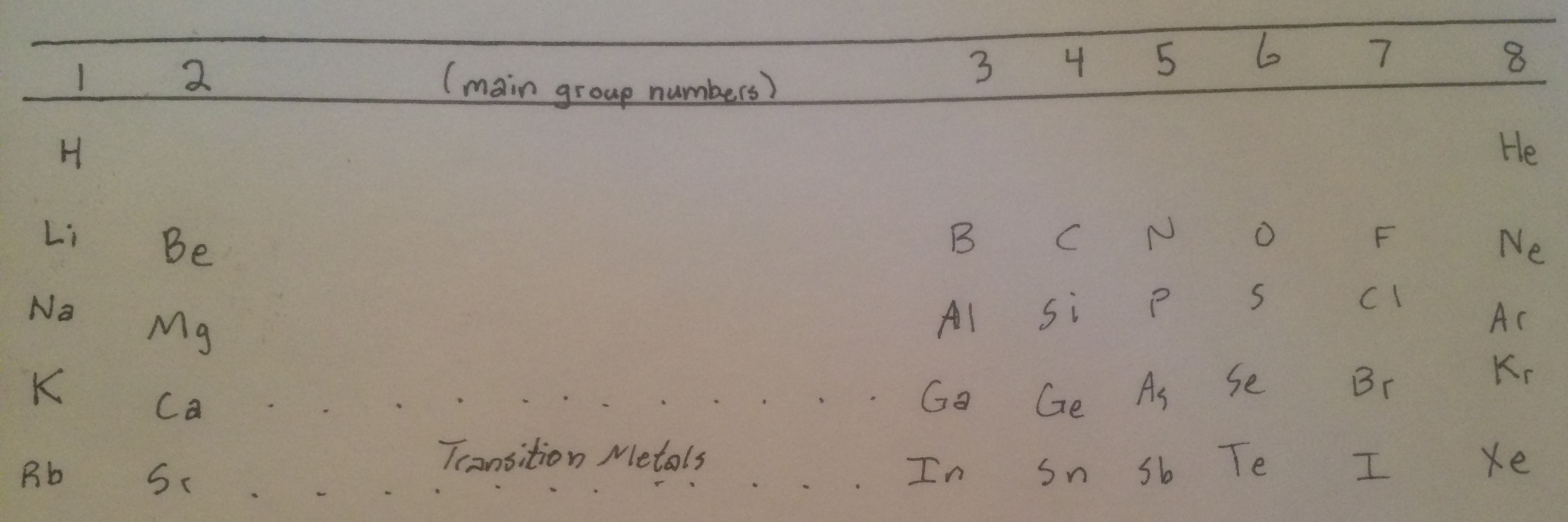### Example Question #105 : High School Chemistry

According to molecular orbital theory, which of the following is not a valid electron configuration?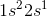All of these are valid electron configurations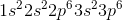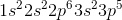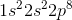Explanation:

We rank the order of orbital filling in order of increasing energy given the probability of an electron occupying a given space. Take into consideration the Aufbau principle, which states that electrons fill electron orbitals from most to least stable. Recall that eight electrons constitutes a full valence shell for all orbitals except for the first one, which has a maximum occupancy of two electrons. The order in which electrons are filled is as follows: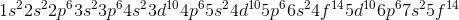### Example Question #106 : High School Chemistry

According to molecular orbital theory, which of the following is not a valid electron configuration?Explanation:

We rank the order of orbital filling in order of increasing energy given the probability of an electron occupying a given space. Take into consideration the Aufbau principle, which states that electrons fill electron orbitals from most to least stable. Recall that eight electrons constitutes a full valence shell for all orbitals except for the first one, which has a maximum occupancy of two electrons. The order in which electrons are filled is as follows:### Example Question #107 : High School Chemistry

According to molecular orbital theory, which of the following is not a valid electron configuration?